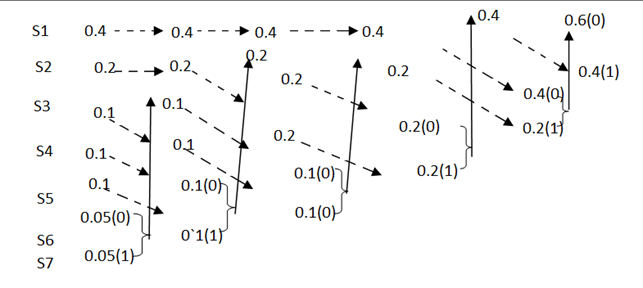Goseeko blog# What is Huffman Coding?

In Huffman Coding the input is having a variable length code. The smallest code is given to the character which occurs the most. The least occurring character has largest code. The concept of prefix code is used here. This means that when code is assigned to any one character that code will not appear again as a prefix of any other code. It is a lossless data compression algorithm.

Firstly, we list the source symbols in the non-increasing order of probabilities

Consider the equation

q = r + (r-1 )

where q = number of source symbols

r = no of different symbols used in the code alphabet

The value of qualify found first and it should be an integer . If it is not an integer we calculate the dummy symbols with zero probabilities. And add them to q to make the quantity an integer. To explain this procedure consider some examples.

## Huffman Coding Method

Given the messages X1,X2,X3,X4,X5 and X6 with respective probabilities of 0.4,0.2,0.2,0.1,0.07 and 0.03. Construct a binary code by applying Huffman encoding procedure . Determine the efficiency and redundancy of the code formed.Now Huffman code is as mentioned below

Now the Average length (L) =   li

L = 0.4 x 1 + 0.2 x 2 + 0.2 x 3 + 0.1 x 4 + 0.07x 5 + 0.03 x 5

L = 2.3 bits/msg symbol.

The value of Entropy is H(s) = =   log 1/Pi

0.4 log 1/0.4 + 0.2 log 1/0.2 + 0.1 log 1/0.1 + 0.07 log 1/0.07 + 0.03 log 1/0.03

H(s) = 2.209 bits /msg symbol.

The Code efficiency will be = nc = H(s) /L = 2.209/2.3 = 96.04%.

Therefore, the efficiency comes out to 96.04%.

Interested in learning about similar topics? Here are a few hand-picked blogs for you!